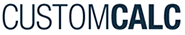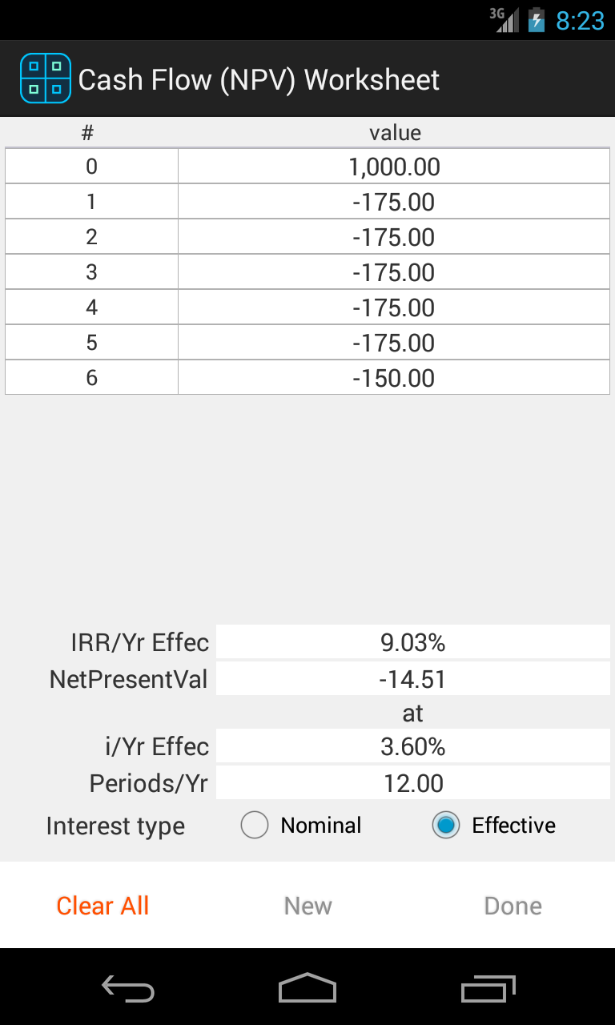﻿ CustomCalc - Android Financial Calculator••# Financial Cash Flow Calculator Tool

In this financial Cash Flow worksheet CustomCalc shows, the Internal Rate of Return (IRR) and the Net Present Value (NPV) based on the Interest rate entered.

The data is entered in the same screen, where you can view, edit, add, or delete values.

When you change any value the worksheet is automatically recalculated to show the real values.

• The Interest Type can be selected from Nominal Interest Rate or Effective Interest Rate
• The interest rate can be edited, as well the number of periods per year, to analyze the results.